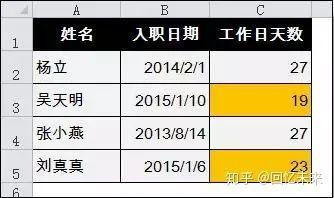# 班主任必看:搞懂这31个excel函数公式,你也可以秒变制表高手!

20 07月

1、Sumproduct函数完成交叉查找

【例】根据姓名和月份，要求从下图上表中查找对应的销量

C14公式

=SUMPRODUCT((A2:A11=A14)*(B1:G1=B14)*B2:G11)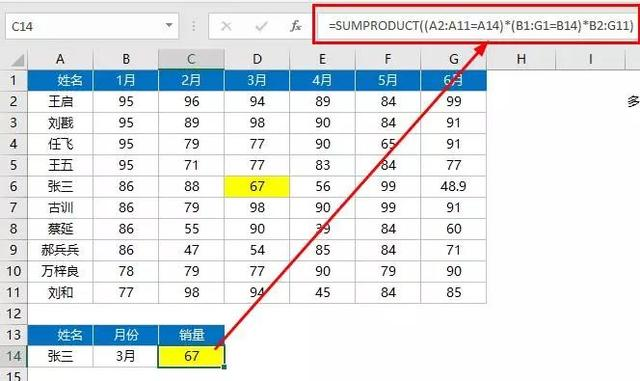2、Vlookup函数隔行求和

Vlookup本是最最常用的查找函数，这次却出现在隔行求和的公式里。

【例】在M2单元格设置公式，隔1列求和

{=SUM(VLOOKUP(A2,A2:K2,ROW(1:6)*2-1,0))}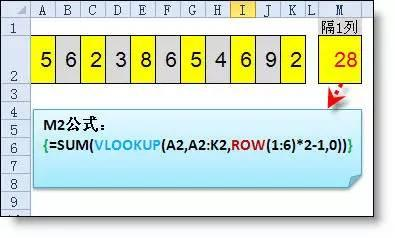{=SUM(VLOOKUP(A2,A2:K2,{2,3,5,6,8,9},0))}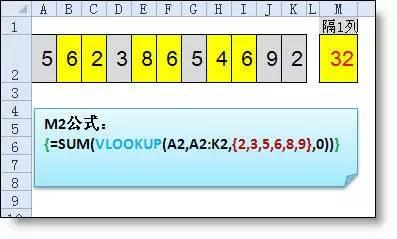3、Min函数判断取值

Min函数不是最小值函数吗怎么抢IF饭碗了。

【例】在D列设置公式计算提成，要求：如果C列数字小于2000则按实际发放，如果大于2000则按2000发放。

=Min(C4,2000)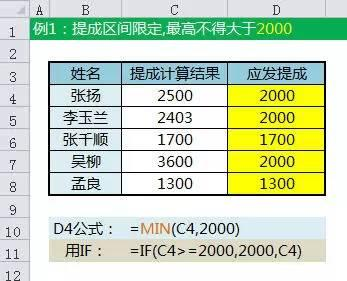=MAX(C4,200)

4、Lookup函数多条件判断

【例】根据下图上表的提成范围，判断后返回对应的提成比率

=LOOKUP(B10,B\$2:C6)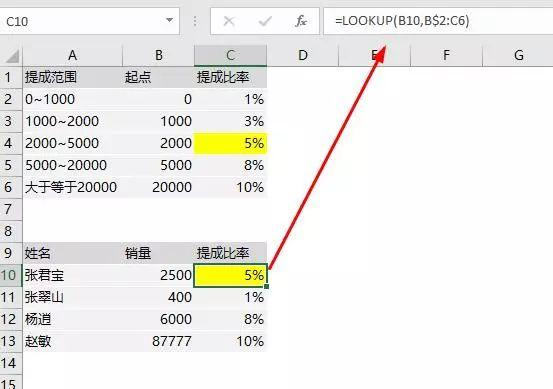1、取绝对值

=ABS(数字)

2、取整

=INT(数字)

3、四舍五入

=ROUND(数字,小数位数)

# 二、判断公式

1、把公式产生的错误值显示为空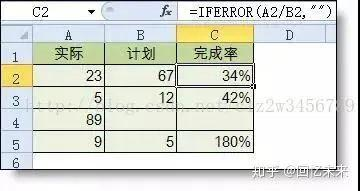2、IF多条件判断返回值公式：C2=IF(AND(A2<500,B2="未到期"),"补款","")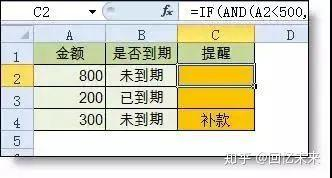# 三、统计公式

1、统计两个表格重复的内容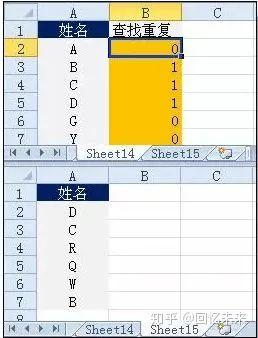2、统计不重复的总人数

=SUMPRODUCT(1/COUNTIF(A2:A8,A2:A8))# 四、求和公式

1、隔列求和公式：H3

=SUMIF(\$A\$2:\$G\$2,H\$2,A3:G3)

=SUMPRODUCT((MOD(COLUMN(B3:G3),2)=0)*B3:G3)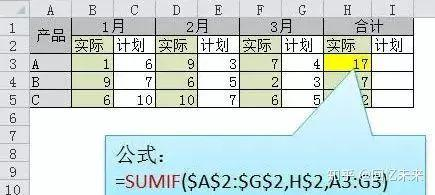2、单条件求和

=SUMIF(A:A,E2,C:C)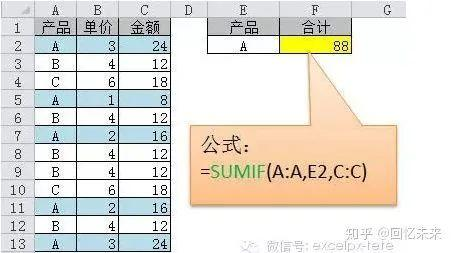3、单条件模糊求和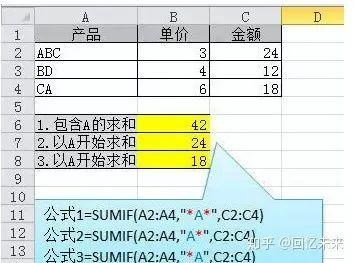4、多条件模糊求和公式：C11=SUMIFS(C2:C7,A2:A7,A11&"*",B2:B7,B11)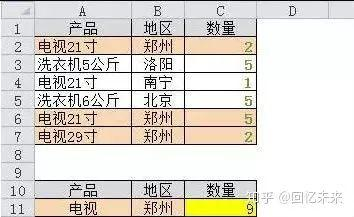5、多表相同位置求和

=SUM(Sheet1:Sheet19!B2)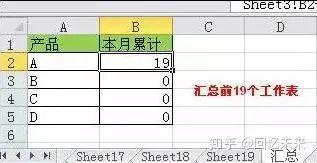6、按日期和产品求和

=SUMPRODUCT((MONTH(\$A\$2:\$A\$25)=F\$1)*(\$B\$2:\$B\$25=\$E2)*\$C\$2:\$C\$25)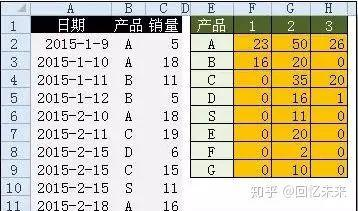# 五、查找与引用公式

1、单条件查找公式

=VLOOKUP(B11,B3:F7,4,FALSE)

2、双向查找公式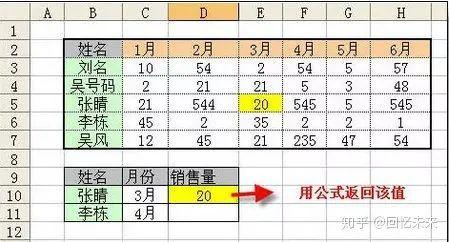3、查找最后一条符合条件的记录。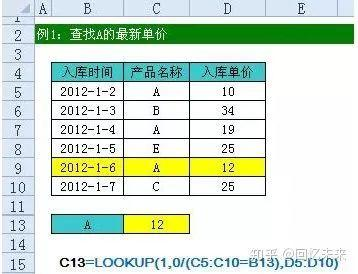4、多条件查找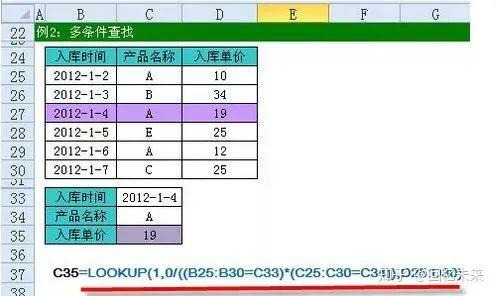5、指定区域最后一个非空值查找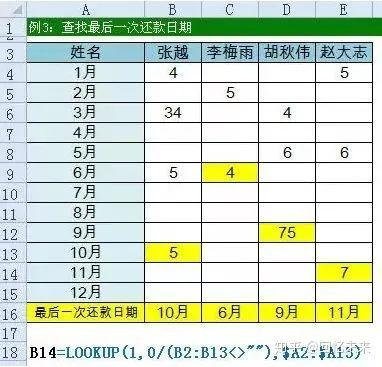6、按数字区域间取对应的值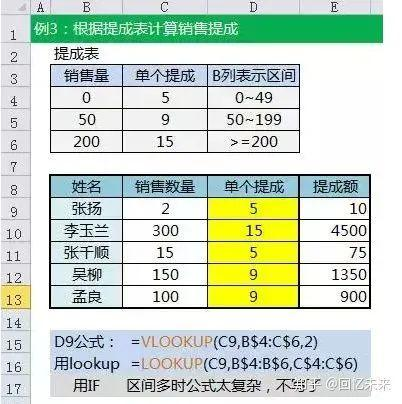# 六、字符串处理公式

1、多单元格字符串合并

=PHONETIC(A2:A7)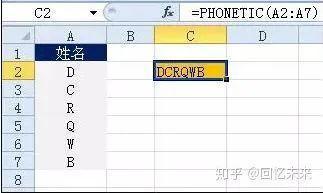2、截取除后3位之外的部分

=LEFT(D1,LEN(D1)-3)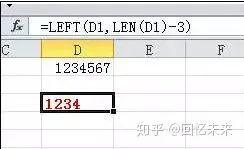3、截取-前的部分

=Left(A1,FIND("-",A1)-1)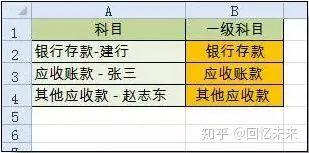4、截取字符串中任一段的公式公式:B1=TRIM(MID(SUBSTITUTE(\$A1," ",REPT(" ",20)),20,20))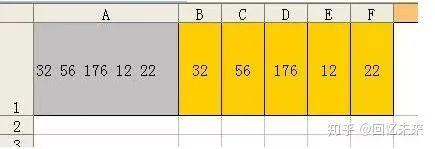5、字符串查找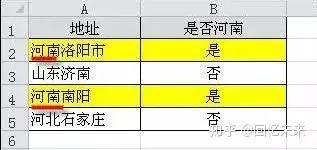6、字符串查找一对多公式：B2=IF(COUNT(FIND({"辽宁","黑龙江","吉林"},A2))=0,"其他","东北")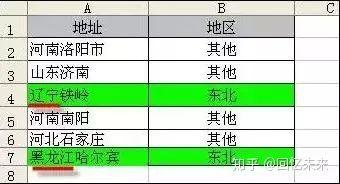# 七、日期计算公式

1、两日期相隔的年、月、天数计算

A1是开始日期（2011-12-1），B1是结束日期(2013-6-10)。计算：相隔多少天？=datedif(A1,B1,"d") 结果：557

datedif函数第3个参数说明：

"Y" 时间段中的整年数。

"M" 时间段中的整月数。

"D" 时间段中的天数。

"MD" 天数的差。忽略日期中的月和年。

"YM" 月数的差。忽略日期中的日和年。

"YD" 天数的差。忽略日期中的年。

2、扣除周末天数的工作日天数

=NETWORKDAYS.INTL(IF(B2<DATE(2015,1,1),DATE(2015,1,1),B2),DATE(2015,1,31),11)